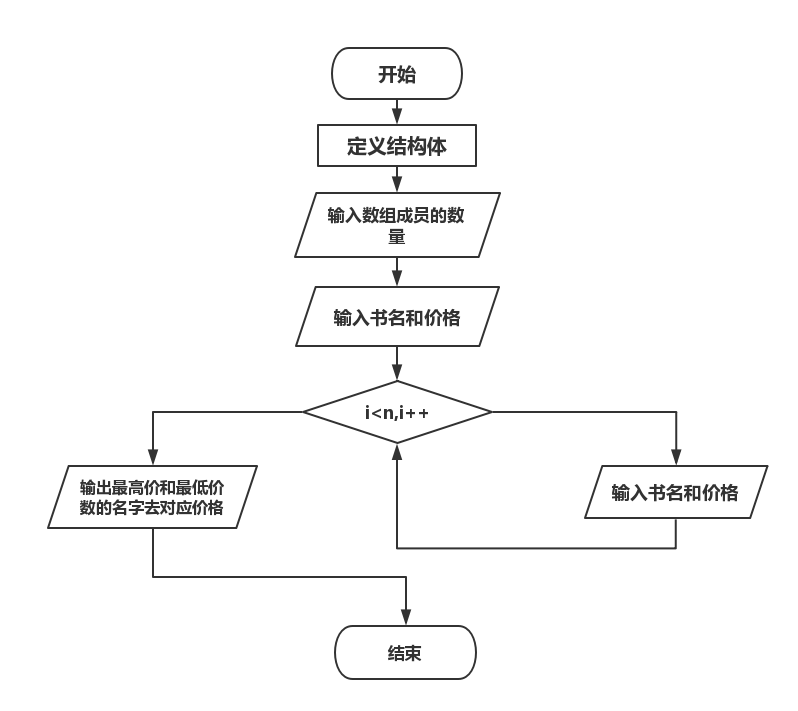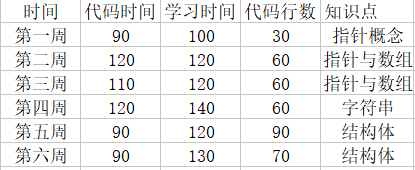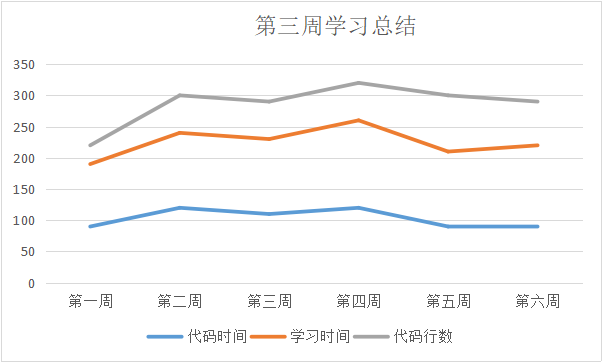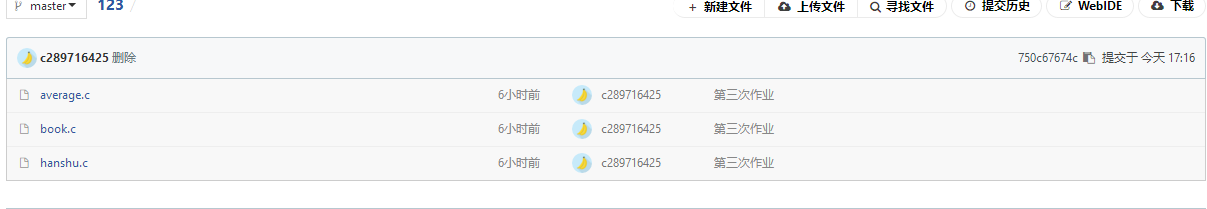# 第三次作业

1、结构成员的引用方法与数组类似在[]运算符中标明要提取的结构体成员放在结构体数组名的后面点运算符的前面，即可提取相应的成员。
2、p->str++中的++加向p中的字符指针str。
3、struct { int len; char str; } p;是个指针定义，p->str++必须加在之后的语句上，这一句不能加，属于语法错误。

1、计算平均成绩：
（1）代码：

#include<stdio.h>
#include<stdlib.h>
struct score
{
char num;
char name;
double s;
};
int main()
{
struct score s;
int i,n;
double average=0;
scanf("%d",&n);
for(i=0;i<n;i++)
{
scanf("%s%s%lf",&s[i].num,&s[i].name,&s[i].s);
}
for(i=0;i<n;i++)
{
average=average+s[i].s;
}
average=average/n;
printf("%.2f\n",average);
for(i=0;i<n;i++)
{
if(s[i].s<average)
{
printf("%s %s\n",s[i].name,s[i].num);

}
}
return 0;
}


（2）设计思路：

（3）流程图：（4）本题遇到的问题：

2、查找书籍
（1）代码：

#include <stdio.h>
#include<stdio.h>
struct book
{
char name;
double price;
}b;
int main()
{
int n,i,min=0,max=0;
scanf("%d",&n);
for(i=0;i<n;i++)
{
scanf("\n");
gets(b[i].name);
scanf("%lf",&b[i].price);
if(b[i].price>b[max].price)
{

max=i;
}
if(b[i].price<b[min].price)
{

min=i;
}
}
printf("%.2lf, %s\n",b[max].price,b[max].name);
printf("%.2lf, %s\n",b[min].price,b[min].name);
return 0;
}


（2）设计思路：

（3）流程图：（4）本题遇到的问题：

（1）学习总结和进度

（2）学习进度表（3）上传coding截图：git地址：https://coding.net/u/c289716425/p/123/git?public=true

posted on 2018-04-23 17:55  CJ丶Madlife  阅读(101)  评论(0编辑  收藏  举报